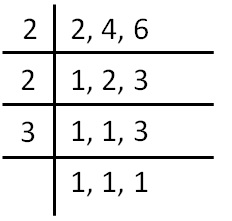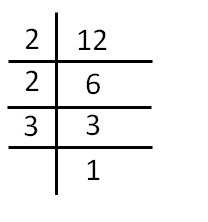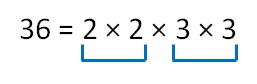Smallest square number divisible by numbers

Chapter 5 Class 8 Squares and Square Roots
Concept wise

Let’s take an example to do it

## What is the smallest square number which is divisible by 2, 4, 6?

We saw that

Smallest number divisible by 2, 4, 6 = LCM of 2, 4, 6

But, we need to find the smallest square number

So,

• We check if LCM is a perfect square
• If its not, then we find the smallest number multiplied to it so that it becomes a perfect square

#### LCM of 2, 4, 6LCM of 2, 4, 6 = 2 × 2 × 3

= 12

Now, checking if 12 is a perfect square or not

#### Checking if 12 is a perfect squareWe see that ,

12 = 2 × 2 × 3

Since 3 does not occur in pairs,

It is not a perfect square

So, we need to find a number to multiply to make pairs

We multiply by 3

So, our number becomes

12 × 3 = 2 × 2 × 3 × 3So, it becomes a perfect square

∴ Smallest square number which is divisible by 2, 4, 6 is 36

Thus, we can write

Smallest square number divisible by 2, 4, 6

= LCM of 2, 4, 6

OR

Multiple of LCM

Learn in your speed, with individual attention - Teachoo Maths 1-on-1 Class